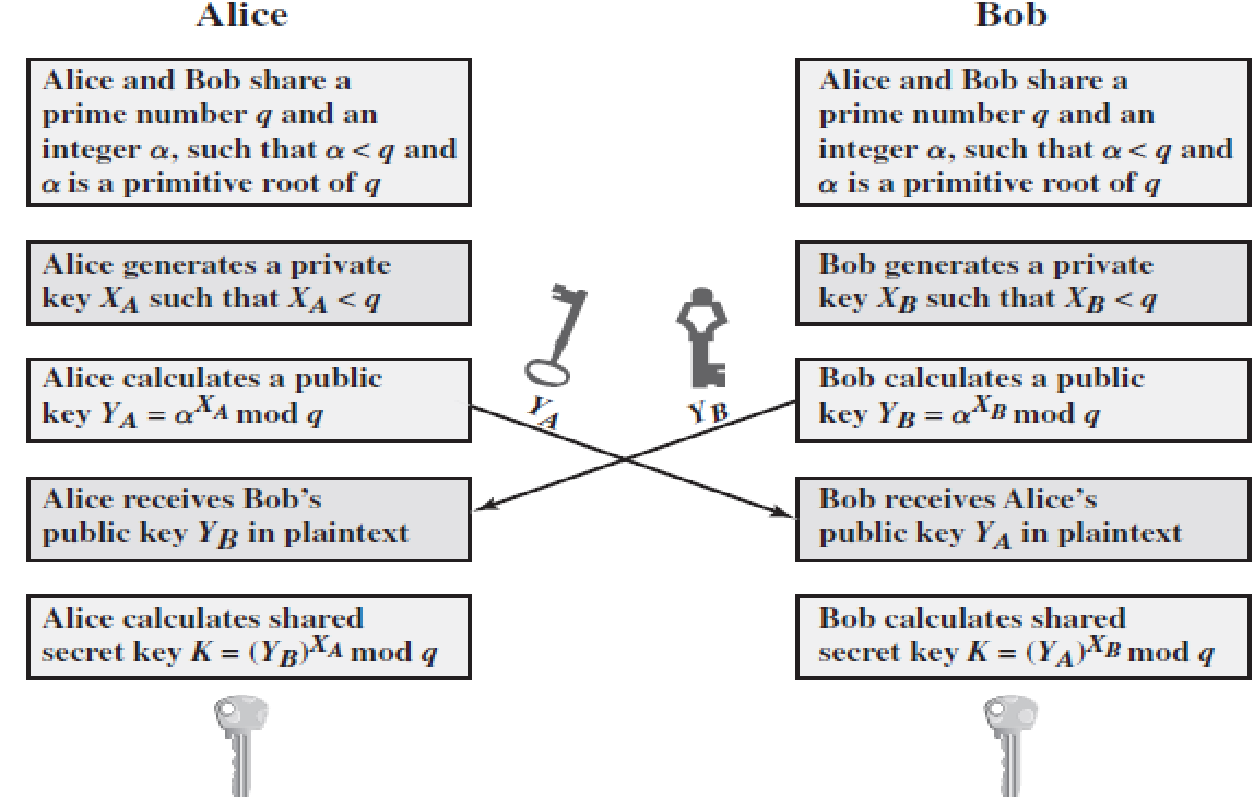# Explain an Diffie-hellman key exchange algorithm?

• Purpose is to enable two users to securely exchange a key that can then be used for subsequent symmetric encryption of messages
• The algorithm is limited to the exchange of secret values
• Its effectiveness depends on the difficulty of computing discrete logarithms• For this scheme, there are two publicly known numbers: a prime number q  and an integer a  that is a primitive root of q
• User A selects a random integer XA < q and computes YA = aXA mod q.
• Similarly, user B independently selects a random integer XB < q and computes YB = aXB mod q.
• Each side keeps the X value private and makes the Y value available publicly to the other side. Thus, XA is A’s private key and YA is A’s corresponding public key, and similarly for B.

User A computes the key as K = (YB)XA mod q    and user B computes the key as K = (YA)XB mod q.

These two calculations of K can be shown to produce identical results:

K = (YB)XA mod q   (this K is the secret key calculated by Alice, since XB is known only to Alice)

= (aXB mod q)XA mod q

= (aXB)XA mod q                 by the rules of modular arithmetic

= aXBXA mod q

= (aXA)XB mod q

= (aXA mod q)XB mod q

= (YA)XB mod q  (here K is secret key calculated by Bob, since XB is known only to Bob)